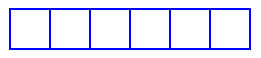Select Page

## Drawing a House

Homework 2, Part 1: Drawing a House

We want to draw a picture of a house with a square on the bottom and a triangle on top. We wrote the script below to do this.## A row of Squares Method 1

Homework 2, Part 2: Row of Squares Method 1

We want to draw a series of squares like the one below. In this homework, we’re going to look at two ways to make a row of squares like this.We’ll start the first method here!

This script draws the shape below.## Row of Squares Method 2

Homework 2, Part 3: Row of Squares Method 2